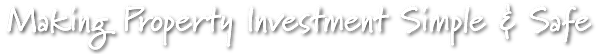## & Growth

• Appraisals / Valuations

A written report of the estimated value of the property,

usually prepared by the valuer.

• Appreciation

An increase in value.

• Basis Point

A measurement of fluctuation of an investment,

equal to 1 / 100 of  one percent.

• Capital Growth (Capital Gain)

Appreciation in the capital or market value of an investment.

The amount by which your investment property has increased

relative to what you paid for it.

## Glossary## & Report's

• Consumer Price Index (CPI)

An index measuring the prices at various times of a selected group of goods and services which typify

those bought by ordinary Australian households.  It allows comparisons of the relative cost of living over time,

and is used as a measure of inflation.

• Diversification

The spreading of investment funds among classes of securities and localities in order to distribute and control

risk.  This is a fundemental law of investing, meaning simply: Don't put all your eggs in one basket.

• Economic Clock

A model (represented by the face of a clock) for depicting the normal sequence of events for share and property

market cycles.  After interest rates fall, the share market rises, followed by commodities, inflation and property.

Interest rates then rise to curb inflation and the cycle goes into decline, before repeating all over again.

Note that not all cities / states are at the sampe place on the clock at the same time.

• Lower Quartile

The price point below which 25 per cent of sales were recorded.

If there were 100 sales in a suburb, the 25th lowest price would be the lower quartile price.

• Median Price

The median house price is the middle price of all sales recorded in a particular suburb, post code, city or state.

If there were 100 sales in a particular suburb, in ascending order, the median would be number 50 on the list.

It's commonly assumed that the median price is the same as the average price, but that's not the case.

To calculate the average, you would add up the 100 sales and divide the total by 100 (the number of sales)

and the number could be quite different to the medium.

• Property Cycle

Property values usually follow a cycle of growth, a slowdown, a bust and an upturn.

History shows this occurs every seven to ten years.

• Rule Of 72

A convenient technique to estimate compound interest rates derived from the fact that a 7.2 per cent return

per year is the rate that will double the value of an investment in ten years.  Similarly, the rate of return needed

to double the value of an investment in a given number of years can be estimated by dividing the number

of years to double into 72.

• Supply & Demand

The number of properties on the market at any given time determines the supply-and-demand equation.

If there are lots of properties on the market, it's a buyers market.  If there are few properties on the market

or those that come on to the market sell quickly, then it's a sellers' market.

• Upper Quartile

The price point below which 75 per cent of sales were recorded.

If there were 100 sales in a suburb, the 25th highest price would be the upper-quartile price.

## Enquiry

Why not book an obligation free

property strategy session?